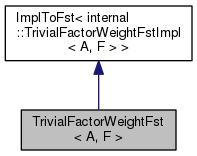TrivialFactorWeightFst< A, F > Class Template Reference

TrivialFactorWeightFst takes as template parameter a FactorIterator as defined above. More...

`#include <trivial-factor-weight.h>`

Inheritance diagram for TrivialFactorWeightFst< A, F >:[legend]
Collaboration diagram for TrivialFactorWeightFst< A, F >:[legend]

## Public Types

typedef A Arc

typedef A::Weight Weight

typedef A::StateId StateId

typedef DefaultCacheStore< ArcStore

typedef Store::State State

typedef internal::TrivialFactorWeightFstImpl< A, F > Impl

## Public Member Functions

TrivialFactorWeightFst (const Fst< A > &fst)

TrivialFactorWeightFst (const Fst< A > &fst, const TrivialFactorWeightOptions< A > &opts)

TrivialFactorWeightFst (const TrivialFactorWeightFst< A, F > &fst, bool copy)

TrivialFactorWeightFst< A, F > * Copy (bool copy=false) const override

void InitStateIterator (StateIteratorData< A > *data) const override

void InitArcIterator (StateId s, ArcIteratorData< A > *data) const override

## Private Member Functions

TrivialFactorWeightFstoperator= (const TrivialFactorWeightFst &fst)=delete

## Friends

class ArcIterator< TrivialFactorWeightFst< A, F > >

class StateIterator< TrivialFactorWeightFst< A, F > >

## Detailed Description

### template<class A, class F> class fst::TrivialFactorWeightFst< A, F >

TrivialFactorWeightFst takes as template parameter a FactorIterator as defined above.

The result of weight factoring is a transducer equivalent to the input whose path weights have been factored according to the FactorIterator. States and transitions will be added as necessary. This algorithm differs from the one implemented in FactorWeightFst in that it does not attempt to push the extra weight forward to the next state: it uses a sequence of "extra" intermediate state, and outputs the remaining weight right away. This ensures that it will always succeed, even for Gallic representations of FSTs that have cycles with more output than input symbols. Note that the code below was modified from factor-weight.h by just search-and-replacing "FactorWeight" by "TrivialFactorWeight".

Definition at line 325 of file trivial-factor-weight.h.

## ◆ Arc

 typedef A Arc

Definition at line 331 of file trivial-factor-weight.h.

## ◆ Impl

 typedef internal::TrivialFactorWeightFstImpl Impl

Definition at line 336 of file trivial-factor-weight.h.

## ◆ State

 typedef Store::State State

Definition at line 335 of file trivial-factor-weight.h.

## ◆ StateId

 typedef A::StateId StateId

Definition at line 333 of file trivial-factor-weight.h.

## ◆ Store

 typedef DefaultCacheStore Store

Definition at line 334 of file trivial-factor-weight.h.

## ◆ Weight

 typedef A::Weight Weight

Definition at line 332 of file trivial-factor-weight.h.

## ◆ TrivialFactorWeightFst() [1/3]

 TrivialFactorWeightFst ( const Fst< A > & fst )
inlineexplicit

Definition at line 338 of file trivial-factor-weight.h.

339  : ImplToFst<Impl>(std::make_shared<Impl>(fst, TrivialFactorWeightOptions<A>())) {}
For an extended explanation of the framework of which grammar-fsts are a part, please see Support for...
Definition: graph.dox:21

## ◆ TrivialFactorWeightFst() [2/3]

 TrivialFactorWeightFst ( const Fst< A > & fst, const TrivialFactorWeightOptions< A > & opts )
inline

Definition at line 341 of file trivial-factor-weight.h.

342  : ImplToFst<Impl>(std::make_shared<Impl>(fst, opts)) {}
For an extended explanation of the framework of which grammar-fsts are a part, please see Support for...
Definition: graph.dox:21

## ◆ TrivialFactorWeightFst() [3/3]

 TrivialFactorWeightFst ( const TrivialFactorWeightFst< A, F > & fst, bool copy )
inline

Definition at line 345 of file trivial-factor-weight.h.

346  : ImplToFst<Impl>(fst, copy) {}
For an extended explanation of the framework of which grammar-fsts are a part, please see Support for...
Definition: graph.dox:21

## ◆ Copy()

 TrivialFactorWeightFst* Copy ( bool copy = `false` ) const
inlineoverride

Definition at line 349 of file trivial-factor-weight.h.

349  {
350  return new TrivialFactorWeightFst<A, F>(*this, copy);
351  }

## ◆ InitArcIterator()

 void InitArcIterator ( StateId s, ArcIteratorData< A > * data ) const
inlineoverride

Definition at line 355 of file trivial-factor-weight.h.

355  {
356  GetMutableImpl()->InitArcIterator(s, data);
357  }

## ◆ InitStateIterator()

 void InitStateIterator ( StateIteratorData< A > * data ) const
inlineoverride

Definition at line 391 of file trivial-factor-weight.h.

392  {
393  data->base = new StateIterator< TrivialFactorWeightFst<A, F> >(*this);
394 }

## ◆ operator=()

 TrivialFactorWeightFst& operator= ( const TrivialFactorWeightFst< A, F > & fst )
privatedelete

## ◆ ArcIterator< TrivialFactorWeightFst< A, F > >

 friend class ArcIterator< TrivialFactorWeightFst< A, F > >
friend

Definition at line 328 of file trivial-factor-weight.h.

## ◆ StateIterator< TrivialFactorWeightFst< A, F > >

 friend class StateIterator< TrivialFactorWeightFst< A, F > >
friend

Definition at line 329 of file trivial-factor-weight.h.

The documentation for this class was generated from the following file: